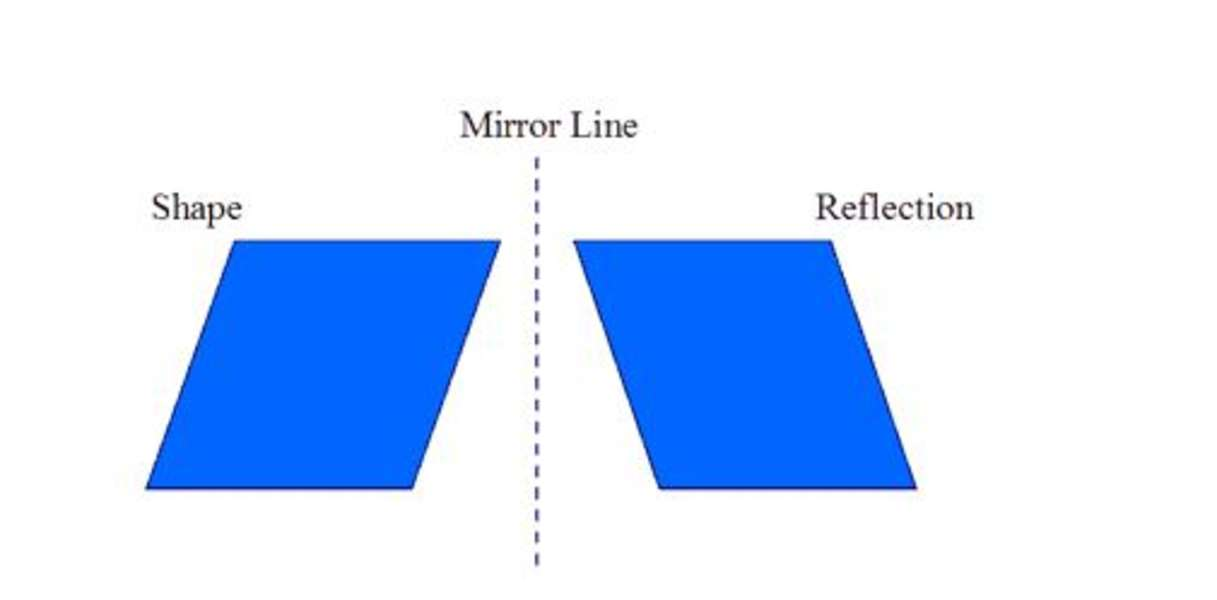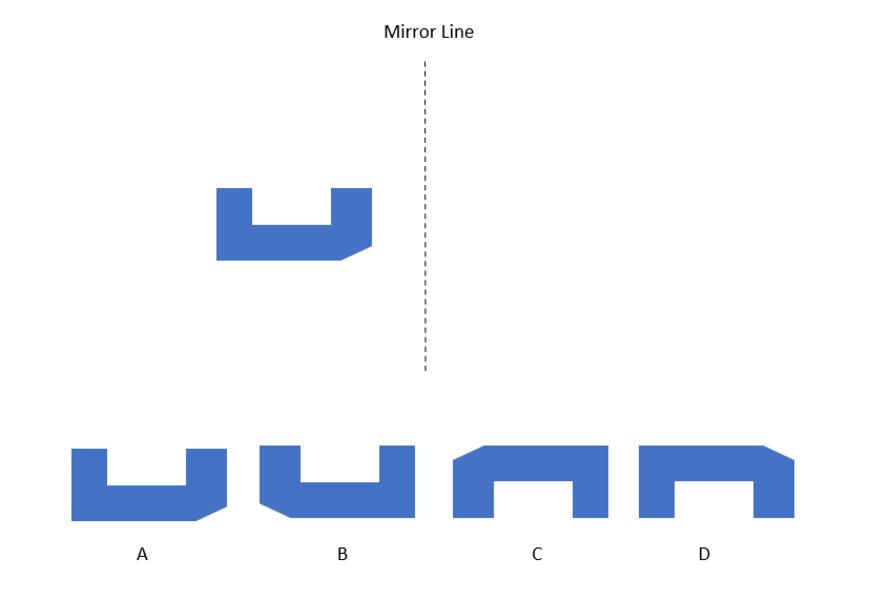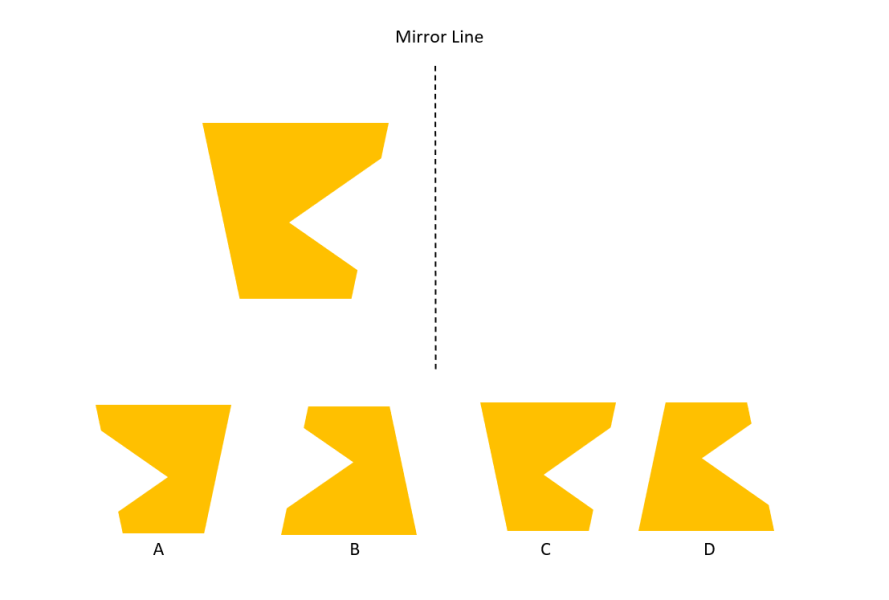Diagrammatic reasoning questions often involve mirror images. Typically, there will be a dotted line representing a mirror and you will be asked to find the corresponding reflected shape.

In the image below, imagine the dotted line and the shape are drawn on a piece of paper. If you were to fold the paper along the dotted line, what position would the shape be in now?

A reflection is a reversed image, so, the correct answer will look like the first shape but backwards.

The corners of the parallelogram are the exact same distance from the mirror, just on the other side. So, one is a reflection of the other.### Example questions

Select the correct reflected shapes.

Example 1:Example 2:### Solutions

Answer for Example 1 = B

Answer for Example 2 = A